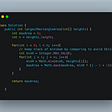# Problem Statement

You are climbing a staircase. It takes n steps to reach the top. Each time you can either take 1 or 2 steps.

# Understanding the Problem

The idea around this problem is pretty straightforward. We want to count unique permutations of steps that will help us reach the nth step.

# High Level Algorithm

DP is an optimization on top of sub-problems (usually recursion), so before we use it, we still need to solve the problem.

1. n == 0: return 1, we reached the nth step.
1. Create an array to store each state you encounter and cache your calculation in the recursive case.
2. Add another base case where you reuse the cache value if you have seen the state.

# Runtime

The brute force recursive solution has a runtime of O(2^N). This is because for every N step, we can make 2 decisions:take 1 step or take 2 steps. This might be okay if N is small, but it exponentially grows larger as N increases.

# Space Complexity

The space complexity of both of our solutions is O(N). In the brute force recursive solution, we are recursively going from 0 to N keeping them in our call stack. Everytime we finish a path, we would pop it out of our callstack so at most it’ll be O(N)

# Implementation

Recursive solution

`class Solution {    public int climbStairs(int n) {        return helper(n);    }       private int helper(int n) {        if (n == 0) {            // base case where we arrived at an answer            return 1;        } else if (n < 0) {            // base case where we went too far            return 0;         } else {            // recursive case where we try taking 1 step            // and 2 steps, adding the unique permuation            // of steps back            return helper(n-1) + helper(n-2);        }    }}`
`class Solution {    private int[] memo;    public int climbStairs(int n) {        // instantiate the datastructure to cache the calculation        // to each of our subproblems. i.e. what step we are on        memo = new int[n+1];        return helper(n);    }       private int helper(int n) {        if (n < 0) {            return 0;        } else if (memo[n] != 0) {            // If we stored something in our cache reuse it and avoid            // recalculating everything            return memo[n];        } else if (n == 0) {            return 1;        } else {            // store our calculation inside our cache so we don't            // have to recalculate it again for memo[n]            memo[n] = helper(n-1) + helper(n-2);            return memo[n];        }    }}`

# Conclusion

To solve Climbing Stairs we need to be able to solve the problem recursively and then apply DP via memoization (caching) to help optimize the runtime.

--

--

--

## More from Josh Chang

Software Engineer by day, side hustler wannabee by night! https://leetdev.io

Love podcasts or audiobooks? Learn on the go with our new app.

## Project Management in 3 Minutes## Python for Data Science: From Scratch (Part I)## Testing of Apache Airflow’s DAGs with docker compose and pytest## Guide to a successful IT startup## How To Send A HTML Form To Email Using PHP## Josh Chang

Software Engineer by day, side hustler wannabee by night! https://leetdev.io

## Leetcode: 84. Largest Rectangle in Histogram## How Software Companies think: Understanding how they function## Steps to solve an algorithm## Dynamic Programming : What, Why and When?